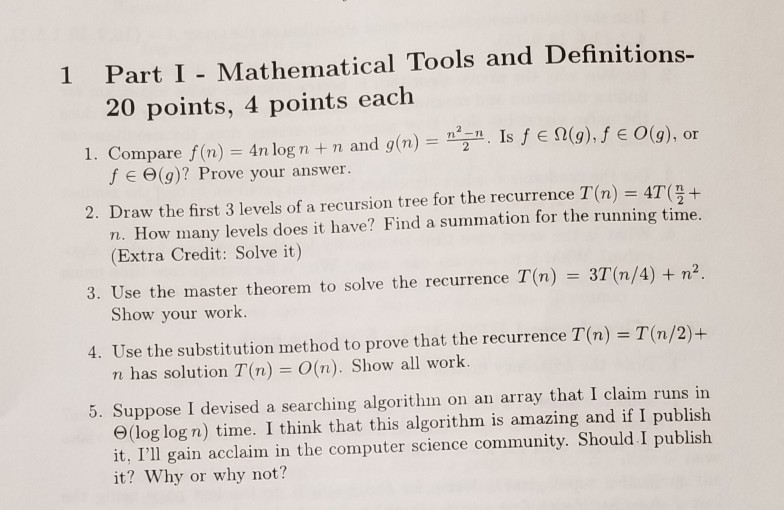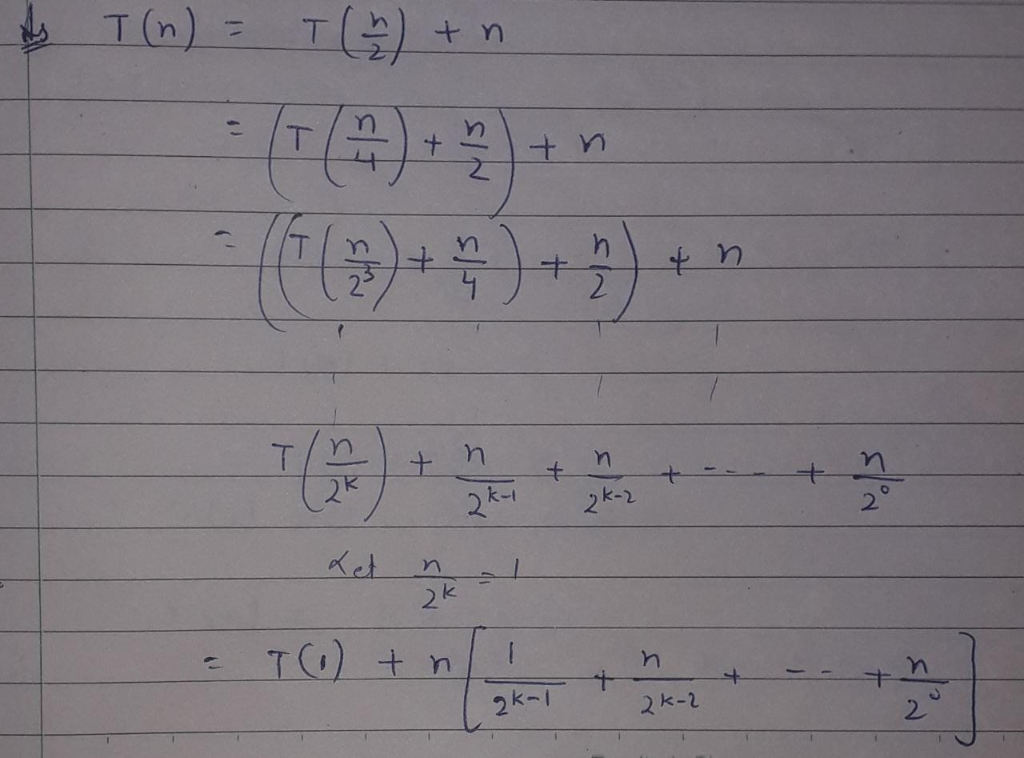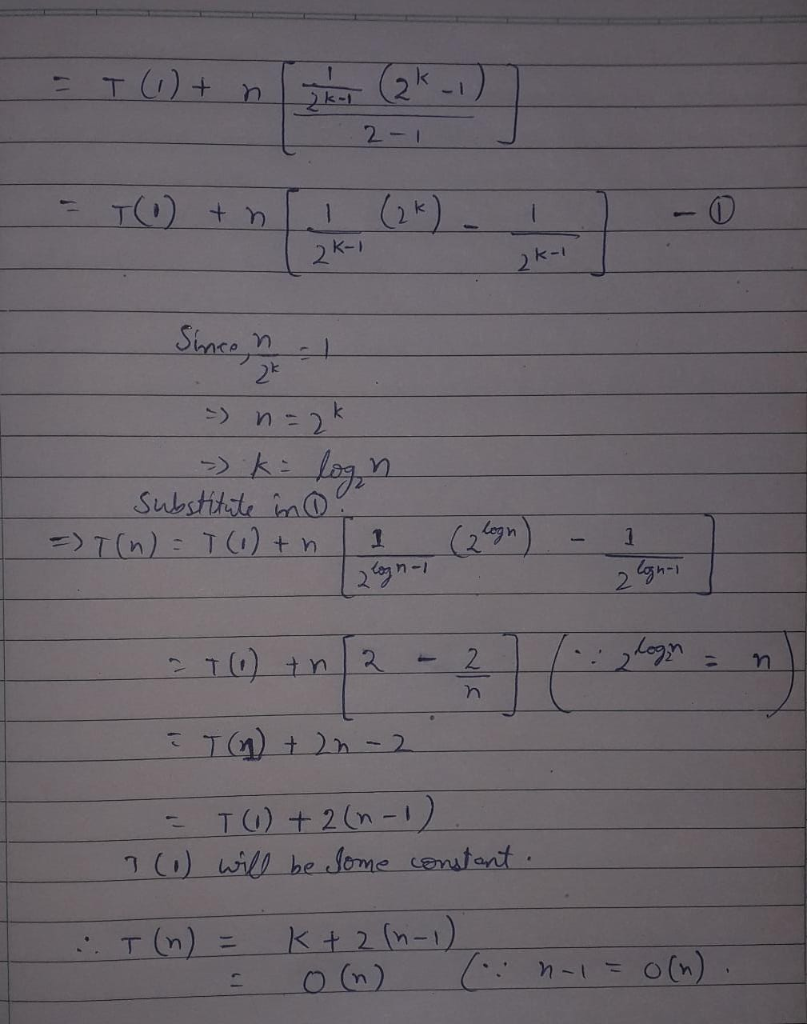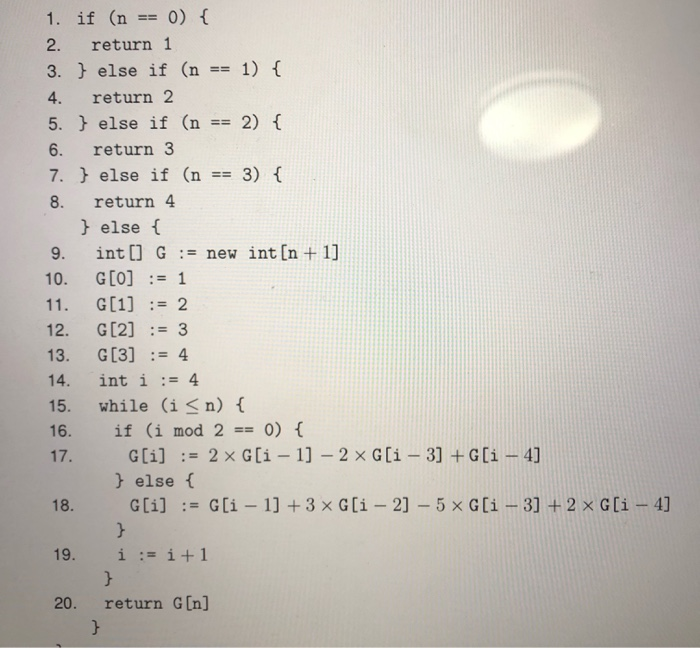Question

Subject: Algorithmsolve only part 4 and 5 please.
need urgent.

Using repetitive substitution, we formed an equation. That equation contains a Geometric progression. We used sum of Geometric Progression to solve that. and formed a big O expression for T(n)Hope this helps
If you have any doubt feel free to comment
Thank You!!

#### Earn Coins

Coins can be redeemed for fabulous gifts.

Similar Homework Help Questions
• ### How to prove G（n）=n+1 in this algorithm？ 1. if (n 0) 2. return 1 3. else if (n1) f 4. return 2 5. else if (n 2) 6. return 3 7. else if (n3) t 8. return 4 else f 9. int OGnew int[n 11 10. G[O]1 1...How to prove G（n）=n+1 in this algorithm？ 1. if (n 0) 2. return 1 3. else if (n1) f 4. return 2 5. else if (n 2) 6. return 3 7. else if (n3) t 8. return 4 else f 9. int OGnew int[n 11 10. G[O]1 12. G3 13. G4 14. int i:-4 15. while (i<n) t 16. if (i mod 20) else ( 20. return G[n] 1. if (n 0) 2. return 1 3. else if (n1) f...